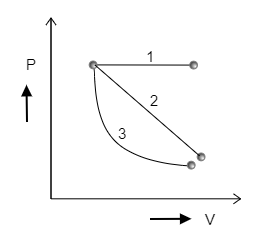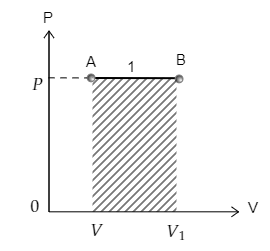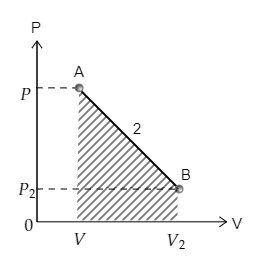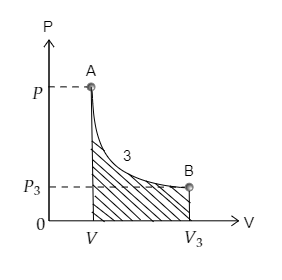Courses
Courses for Kids
Free study material
Offline Centres
MoreLast updated date: 29th Nov 2023
Total views: 343.2k
Views today: 5.43k

# Determine for which of the following three P-V diagrams shown in the figure is the work done the minimum.A) 1B) 2C) 3D) Cannot be determined.Verified
343.2k+ views
Hint: In a graph between the pressure of the gas and the volume of the gas, the area under the curve which represents the transition of the gas from one state to another will determine the work done by the gas. So a smaller area under the curve will indicate that lesser work is done to attain the transition of the gas.

Step 1: Sketching each curve individually and marking the area under the curves to determine which curve involves minimum work.
The curve 1 depicting the transition from point A to point B is sketched below. The area under the curve 1 is also marked. The pressure at A is $P$ and volume is $V$ while the pressure and volume at B are ${P_1} = P$ and ${V_1}$ respectively.Now the curve 2 depicting the transition from point A to point B is sketched below. The area under the curve 2 is also marked. The pressure at A is $P$ and volume is $V$ while the pressure and volume at B are ${P_2}$ and ${V_2}$ respectively.Finally, curve 3 depicting the transition from point A to point B is sketched below. The area under the curve 3 is also marked. The pressure at A is $P$ and volume is $V$ while the pressure and volume at B are ${P_3}$ and ${V_3}$ respectively.Now on observing the areas under each curve we realize that the area under curve 3 is minimum. Then the work done will also be minimum for curve 3.

Therefore, the correct option is C.

Note:
The work done by the gas depends on the change in the volume $V$ and the change in the pressure $P$ of the gas as $W = \Delta \left( {PV} \right)$. The given curves depict an increase in the volume of the gas. For curve 1, the pressure remained constant as the volume increased. While for curve 2 and curve 3, the pressure dropped with the increase in volume. Curve 1 describes an isobaric process as the pressure remained constant.